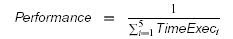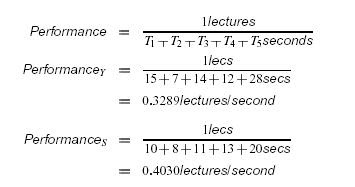# Funny twist with compression and performance - Interview Question

The organization Scholastic Lecture Expert Productivity Testers (SLEPT), has defined a standardized algorithm to compress digital-video lectures by removing irrelevant material. The company you work for, Yawn Inc, is developing a circuit that implements this compression algorithm. A competing company, Snore Co, has just released their own circuit that also implements the standardized algorithm.

SLEPT has established a benchmark of five lectures to use for comparing circuits that implement the compression algorithm.
The CEO of your company, Dr. Owsy has asked you to calculate the price at which you should sell the Yawn circuit such that you can advertise to consumers that the price/performance ratio for the Yawn circuit is 10% better than the Snore circuit.

You evaluate a Yawn circuit and a Snore circuit in your lab, and gather the following data. Unfortunately, you fall asleep on the backspace button, deleting the information for the length of lectures after compression for the Yawn circuit.

Collect the below data...1. Define “performance” for circuits that run the SLEPT compression algorithm. Briefly justify your definition.
2. Assuming that the selling price of Snore Co’s circuit is \$150, compute the selling price for the Yawn circuit that would make its price/performance ratio 10% better than the Snore circuit. If you do not have enough information to calculate the selling price: explain how you could obtain the missing information and show your equation that uses the missing information to calculate the selling price.
Solution:
1. Performance is work/time.The work that the circuit does is to compress lecures. The SLEPT algorithm is standardized. All circuits that implement the algorithm will produce the same results (e.g. same amount of compression). Hence, all circuits do the same amount of work.The time taken to do the work is the “Execution time for circuit”. Because we have a benchmark, the execution time to compare is the time to run the benchmark, that is, compress the five lectures in the benchmark.
2.A better price/performance ratio means that the price is lower or the performance is greater: the lower the ratio the better the ratio.

Starting with the formula for “a is n% more than b”: we get the followingDifficulty:
Medium